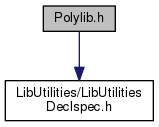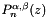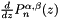Nektar++
Polylib.h File Reference
#include <LibUtilities/LibUtilitiesDeclspec.h>
Include dependency graph for Polylib.h:This graph shows which files directly or indirectly include this file:Go to the source code of this file.

## Namespaces

Polylib
The namespace associated with the the Polylib library (Polylib introduction)

#define H_PLYLIB

## Functions

void Polylib::zwgj (double *z, double *w, const int np, const double alpha, const double beta)
Gauss-Jacobi zeros and weights. More...

void Polylib::zwgrjm (double *z, double *w, const int np, const double alpha, const double beta)
Gauss-Radau-Jacobi zeros and weights with end point at z=-1. More...

void Polylib::zwgrjp (double *z, double *w, const int np, const double alpha, const double beta)
Gauss-Radau-Jacobi zeros and weights with end point at z=1. More...

void Polylib::zwglj (double *z, double *w, const int np, const double alpha, const double beta)
Gauss-Lobatto-Jacobi zeros and weights with end point at z=-1,1. More...

void Polylib::zwgk (double *z, double *w, const int npt, const double alpha, const double beta)
Gauss-Kronrod-Jacobi zeros and weights. More...

void Polylib::zwrk (double *z, double *w, const int npt, const double alpha, const double beta)

void Polylib::zwlk (double *z, double *w, const int npt, const double alpha, const double beta)
Gauss-Lobatto-Kronrod-Jacobi zeros and weights. More...

void Polylib::JacZeros (const int n, double *a, double *b, const double alpha, const double beta)
Zero and Weight determination through the eigenvalues and eigenvectors of a tridiagonal. More...

void Polylib::Dgj (double *D, const double *z, const int np, const double alpha, const double beta)
Compute the Derivative Matrix and its transpose associated. More...

void Polylib::Dgrjm (double *D, const double *z, const int np, const double alpha, const double beta)
Compute the Derivative Matrix and its transpose associated. More...

void Polylib::Dgrjp (double *D, const double *z, const int np, const double alpha, const double beta)
Compute the Derivative Matrix associated with the. More...

void Polylib::Dglj (double *D, const double *z, const int np, const double alpha, const double beta)
Compute the Derivative Matrix associated with the. More...

double Polylib::hgj (const int i, const double z, const double *zgj, const int np, const double alpha, const double beta)
Compute the value of the i th Lagrangian interpolant through. More...

double Polylib::hgrjm (const int i, const double z, const double *zgrj, const int np, const double alpha, const double beta)
Compute the value of the i th Lagrangian interpolant through the. More...

double Polylib::hgrjp (const int i, const double z, const double *zgrj, const int np, const double alpha, const double beta)
Compute the value of the i th Lagrangian interpolant through the. More...

double Polylib::hglj (const int i, const double z, const double *zglj, const int np, const double alpha, const double beta)
Compute the value of the i th Lagrangian interpolant through the. More...

void Polylib::Imgj (double *im, const double *zgj, const double *zm, const int nz, const int mz, const double alpha, const double beta)
Interpolation Operator from Gauss-Jacobi points to an. More...

void Polylib::Imgrjm (double *im, const double *zgrj, const double *zm, const int nz, const int mz, const double alpha, const double beta)
Interpolation Operator from Gauss-Radau-Jacobi points. More...

void Polylib::Imgrjp (double *im, const double *zgrj, const double *zm, const int nz, const int mz, const double alpha, const double beta)
Interpolation Operator from Gauss-Radau-Jacobi points. More...

void Polylib::Imglj (double *im, const double *zglj, const double *zm, const int nz, const int mz, const double alpha, const double beta)
Interpolation Operator from Gauss-Lobatto-Jacobi points. More...

void Polylib::jacobfd (const int np, const double *z, double *poly_in, double *polyd, const int n, const double alpha, const double beta)
Routine to calculate Jacobi polynomials,, and their first derivative,. More...

void Polylib::jacobd (const int np, const double *z, double *polyd, const int n, const double alpha, const double beta)
Calculate the derivative of Jacobi polynomials. More...

## Macro Definition Documentation

 #define H_PLYLIB

Definition at line 166 of file Polylib.h.